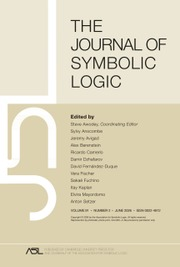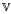Home
Hostname: page-component-59b7f5684b-s82fj Total loading time: 0.778 Render date: 2022-10-01T18:59:38.470Z Has data issue: true Feature Flags: { "shouldUseShareProductTool": true, "shouldUseHypothesis": true, "isUnsiloEnabled": true, "useRatesEcommerce": false, "displayNetworkTab": true, "displayNetworkMapGraph": false, "useSa": true } hasContentIssue trueThe Journal of Symbolic Logic

# Quasi-subtractive varieties

Published online by Cambridge University Press:  12 March 2014

## Abstract

Varieties like groups, rings, or Boolean algebras have the property that, in any of their members, the lattice of congruences is isomorphic to a lattice of more manageable objects, for example normal subgroups of groups, two-sided ideals of rings, filters (or ideals) of Boolean algebras. Abstract algebraic logic can explain these phenomena at a rather satisfactory level of generality: in every member A of a τ-regular varietythe lattice of congruences of A is isomorphic to the lattice of deductive filters on A of the τ-assertional logic of. Moreover, ifhas a constant 1 in its type and is 1-subtractive, the deductive filters on Aof the 1-assertional logic ofcoincide with the-ideals of A in the sense of Gumm and Ursini, for which we have a manageable concept of ideal generation.

However, there are isomorphism theorems, for example, in the theories of residuated lattices, pseudointerior algebras and quasi-MV algebras that cannot be subsumed by these general results. The aim of the present paper is to appropriately generalise the concepts of subtractivity and τ-regularity in such a way as to shed some light on the deep reason behind such theorems. The tools and concepts we develop hereby provide a common umbrella for the algebraic investigation of several families of logics, including substructural logics, modal logics, quantum logics, and logics of constructive mathematics.

Type
Research Article
Information
The Journal of Symbolic Logic , December 2011 , pp. 1261 - 1286
Copyright © Association for Symbolic Logic 2011

## Access options

Get access to the full version of this content by using one of the access options below. (Log in options will check for institutional or personal access. Content may require purchase if you do not have access.)

## References

#### REFERENCES

Aglianò, P. and Ursini, A., On subtractive varieties II: General properties, Algebra Universalis, vol. 36 (1996), pp. 222259.CrossRefGoogle Scholar
Aglianò, P. and Ursini, A., On subtractive varieties III: From ideals to congruences, Algebra Universalis, vol. 37 (1997), pp. 296333.CrossRefGoogle Scholar
Aglianò, P. and Ursini, A., On subtractive varieties IV: Definability of principal ideals, Algebra Universalis, vol. 38 (1997), pp. 355389.CrossRefGoogle Scholar
Ardeshir, M. and Ruitenburg, W., Basic propositional calculus I, Mathematical Logic Quarterly, vol. 44 (1998), pp. 317343.CrossRefGoogle Scholar
Barbour, G. D. and Raftery, J. G., Quasivarieties of logic, regularity conditions and parameterized algebraization, Studio Logica, vol. 74 (2003), pp. 99152.CrossRefGoogle Scholar
Blok, W. J. and Pigozzi, D., Algebraizable logics, Memoirs of the AMS, no. 396, American Mathematical Society, Providence, RI, 1989.Google Scholar
Blok, W. J. and Pigozzi, D., On the structure of varieties with equationally definable principal congruences IV, Algebra Universalis, vol. 31 (1994), pp. 135.CrossRefGoogle Scholar
Blok, W. J. and Raftery, J. G., Ideals in quasivarieties of algebras, Models, algebras and proofs (Caicedo, X. and Montenegro, C. H., editors), Leecture Notes in Pure and Applied Mathematics, Dekker, 1999. pp. 167186.Google Scholar
Blok, W. J. and Raftery, J. G., Assertionally equivalent quasivarieties, International Journal of Algebra and Computation, vol. 18 (2008), pp. 589681.CrossRefGoogle Scholar
Bou, F., Paoli, F., Ledda, A., and Freytes, H., On some properties of quasi-MV algebras andquasi-MV algebras. Part II, Soft Computing, vol. 12 (2008), pp. 341352.CrossRefGoogle Scholar
Burris, S. and Sankappanavar, H. P., A course in universal algebra, Graduate Texts in Mathematics, no. 78, Springer, 1981.CrossRefGoogle Scholar
Chajda, I., Congruence properties of algebras in nilpotent shifts of varieties, General algebra and discrete mathematics (Denecke, K. and Lüders, O., editors), Heidermann, Berlin, 1995, pp. 3546.Google Scholar
Chajda, I., Normally presented varieties, Algebra Universalis, vol. 34 (1995), pp. 327335.CrossRefGoogle Scholar
Chajda, I., Halas, R., Kühr, J., and Vanzurova, A., Normalization of MV algebras, Mathematica Bohemica, vol. 130 (2005), no. 3, pp. 283300.Google Scholar
Czelakowski, J., Equivalential logics I, Studia Logica, vol. 45 (1981), pp. 227236.CrossRefGoogle Scholar
Darnel, M. R., Theory of lattice ordered groups, Dekker, New York, 1995.Google Scholar
Duda, J., Arithmelicity at 0, Czechoslovak Mathematical Journal, vol. 37 (1987), pp. 197206.Google Scholar
Epstein, G. and Horn, A., Logics which are characterised by subresiduated lattices, Zeitschrift für mathematische Logik und Grundlagen der Mathematik, vol. 22 (1976), pp. 199210.CrossRefGoogle Scholar
Font, J. M., Jansana, R., and Pigozzi, D., A survey of abstract algebraic logic, Studia Logica, vol. 74 (2003), pp. 1397.CrossRefGoogle Scholar
Galatos, N., Jipsen, P., Kowalski, T., and Ono, H., Residuated lattices: An algebraic glimpse on substructurai logics, Logic and the Foundations of Mathematics, vol. 151, Elsevier, Amsterdam, 2007.Google Scholar
Galatos, N. and Tsinakis, C., Generalized MV algebras, Journal of Algebra, vol. 283 (2005), no. 1, pp. 254291.CrossRefGoogle Scholar
Graczynska, E., On normal and regular identities, Algebra Universalis, vol. 27 (1990), pp. 387397.CrossRefGoogle Scholar
Grätzer, G., Lakser, H., and Płonka, J., Joins and direct products of equational classes, Canadian Mathematical Bulletin, vol. 12 (1969), pp. 741744.CrossRefGoogle Scholar
Gumm, H. P. and Ursini, A., Ideals in universal algebra, Algebra Universalis, vol. 19 (1984), pp. 4554.CrossRefGoogle Scholar
Jónsson, B. and Tsinakis, C., Products of classes of residuated structures, Studia Logica, vol. 77 (2004), pp. 267292.CrossRefGoogle Scholar
Klunder, B., Representable pseudo-interior algebras, Algebra Universalis, vol. 40 (1998), pp. 177188.CrossRefGoogle Scholar
Kowalski, T., Semisimplicity, EDPC and discriminator varieties of residuated lattices, Studia Logica, vol. 77 (2005), pp. 255265.CrossRefGoogle Scholar
Kowalski, T. and Paoli, F., On some properties of quasi-MV algebras andquasi-MV algebras. Part III, Reports on Mathematical Logic, vol. 45 (2010), pp. 161199.Google Scholar
Kowalski, T. and Paoli, F., Joins and subdirect products of varieties, Algebra Universalis, vol. 65 (2011), pp. 371391.CrossRefGoogle Scholar
Ledda, A., Konig, M., Paoli, F., and Giuntini, R., MV algebras and quantum computation, Studia Logica, vol. 82 (2006), pp. 245270.CrossRefGoogle Scholar
Mal'cev, A. I., On the general theory of algebraic systems, Matematicheskii Sbornik (N.S.), vol. 35(77) (1954), no. 1, pp. 320.Google Scholar
Mc Kenzie, R., An algebraic version of categorical equivalence for varieties and more general algebraic categories, Logic and algebra: Proceedings of the Magari conference (Ursini, A. and Aglianó, P., editors), Dekker, New York, 1996, pp. 211243.Google Scholar
Mc Kenzie, R., Mc Nulty, G., and Taylor, W., Algebras, lattices, varieties, vol. 1, Wadsworth & Brooks/Cole, Monterey, California, 1987.Google Scholar
Mel'nik, L. I., Nilpotent shifts of varieties, Mathematical Notes, vol. 14 (1973), pp. 692696.CrossRefGoogle Scholar
Paoli, F., Ledda, A., Giuntini, R., and Freytes, H., On some properties of quasi-MV algebras andquasi-MV algebras. Part I, Reports on Mathematical Logic, vol. 44 (2008), pp. 5385.Google Scholar
Sambin, G. and Vaccaro, V., Topology and duality in modal logic, Annals of Pure and Applied Logic, vol. 37 (1988), pp. 249296.CrossRefGoogle Scholar
Spinks, M. and Veroff, R., Constructive logic with strong negation is a substructural logic I, Studia Logica, vol. 88 (2008), pp. 325348.CrossRefGoogle Scholar
Spinks, M. and Veroff, R., Constructive logic with strong negation is a substructural logic II, Studia Logica, vol. 89 (2008), pp. 401425.CrossRefGoogle Scholar
Ursini, A., On subtractive varieties I, Algebra Universalis, vol. 31 (1994), pp. 204222.CrossRefGoogle Scholar
Vakarelov, D., Notes on N-lattices and constructive logic with strong negation, Studia Logica, vol. 36 (1977), pp. 109125.CrossRefGoogle Scholar
van Alten, C. J., An algebraic study of residuated ordered monoids and logics without exchange and contraction, Ph.D. thesis, University of Natal, 1998.Google Scholar
Werner, H., Discriminator algebras, Akademie Verlag, Berlin, 1978.Google Scholar
10
Cited by

# Save article to Kindle

To save this article to your Kindle, first ensure coreplatform@cambridge.org is added to your Approved Personal Document E-mail List under your Personal Document Settings on the Manage Your Content and Devices page of your Amazon account. Then enter the ‘name’ part of your Kindle email address below. Find out more about saving to your Kindle.

Note you can select to save to either the @free.kindle.com or @kindle.com variations. ‘@free.kindle.com’ emails are free but can only be saved to your device when it is connected to wi-fi. ‘@kindle.com’ emails can be delivered even when you are not connected to wi-fi, but note that service fees apply.

Find out more about the Kindle Personal Document Service.

Quasi-subtractive varieties
Available formats
×

# Save article to Dropbox

To save this article to your Dropbox account, please select one or more formats and confirm that you agree to abide by our usage policies. If this is the first time you used this feature, you will be asked to authorise Cambridge Core to connect with your Dropbox account. Find out more about saving content to Dropbox.

Quasi-subtractive varieties
Available formats
×

# Save article to Google Drive

To save this article to your Google Drive account, please select one or more formats and confirm that you agree to abide by our usage policies. If this is the first time you used this feature, you will be asked to authorise Cambridge Core to connect with your Google Drive account. Find out more about saving content to Google Drive.

Quasi-subtractive varieties
Available formats
×
×